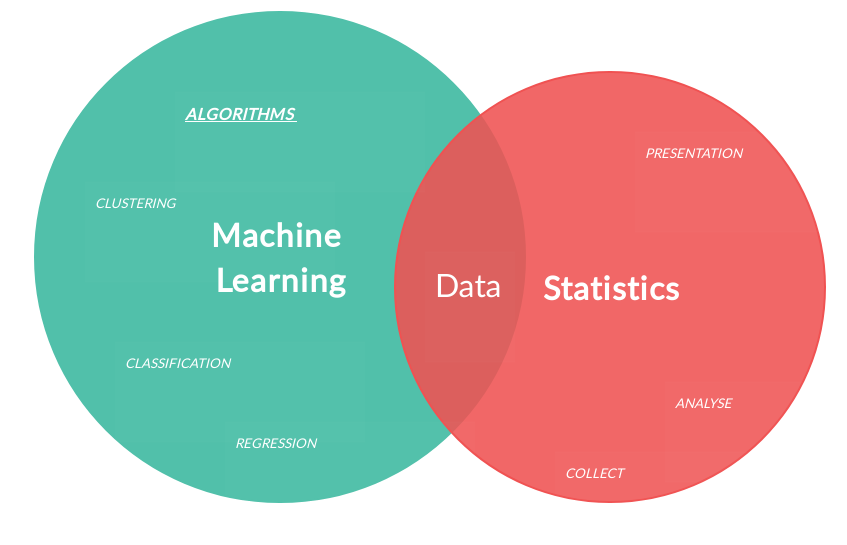The differences between Statistics and Machine Learning.

Things can have similarities and differences when they are not the same. That’s the thing between Statistics and Machine Learning, they are not the same.

Most of people can be confused about it, because there’s a common thought that the difference between Statistics and Machine Learning is their purpose. It is, but at the same time, it’s not.

Related course:

## Statistics and Machine Learning comparison

To understand it better, we must know that Statistics is a branch of Mathematics and Machine Learning is a branch of Artificial Intelligence.

So, what are these things?

• Statistics deals with data collection, organization, analysis, interpretation and presentation.

• Machine Learning uses algorithms to learn and improve from experience.

Basically, Statistics and Machine Learning have data in common.
Data can have qualitative or quantitative variables, both use those variables.### What is Data Science?

There’s also something we must know at this point, Data Science. Data Science uses powerful hardware’s, powerful programming systems and efficient algorithms to solve problems.

Data Science is the method to work with Data. You don’t need a computer to do Statistics, but you needed it to do Data Science.### What is a Statistical Model?

Last, but not least, there’s Statistical Model. It is the process of using data to construct a mathematical or algorithmic device to measure the probability of some observation.

### Differences between Statistics and Machine Learning

Statistics, Statistical Model, Machine Learning, Data Science and Data can have similarities but the difference is there.

1. Machine learning is built upon a Statistical Framework. It involves data and data has to be described using a Statistical Framework.

2. Statistics deal with data, Machine Learning uses Data to Train and Test by itself.

3. Statistics are data points and Machine Learning is prediction.

4. Statistics types are Forecasting continuous variables, Regression and classification. Machine Learning types are supervised and unsupervised learning.

5. The Input-Output of Statistics are the Data Points and of Machine Learning are Features and Labels.

6. Statistics is use for the correlation between the data points, univariate and multivariable and Machine Learning is use for hypothesis.

7. Statistics require Mathematics knowledge and Machine Learning requires Mathematics and Algorithms.

8. Statistics applications are Descriptive statistics, finding patterns and outliers in the data. Machine Learning applications are Weather Forecast, Topic Modeling and Predictive modeling.

9. Statistics stands out Derivatives and Probabilities, Machine Learning stands out Predominant algorithms and concepts like Neural Networks.

10. Working with Statistics we understand concepts like Covariance, Univariate, Multivariate, Estimators, P-values and Root-Mean-Square Deviation.

Working with Machine Learning we understand concepts like Linear Regression, Random Forest, Support vector machine and Neural Networks.

Due to the abundance of data humanity has access to, Machine Learning can more useful, but we can’t forget Machine Learning would not exist without statistics.

It can’t be a Statistics vs. Machine Learning to decide anything, the purpose will decide for you.